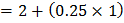# What is the potential drop between points A and C in the following circuit? Resistances 1and 2represent the internal resistance of the respective cellsa) 1.75V b) 2.25V c)d)## Question ID - 151471 :- What is the potential drop between points A and C in the following circuit? Resistances 1and 2represent the internal resistance of the respective cellsa) 1.75V b) 2.25V c)d)3537

(b)

Emf’sandare opposing each other. Sinceso, current will move from right to left.Current in circuitThe potential drop between points A and C isNext Question :

A long straight wire of a circular cross section (radius) carries a steady currentand the currentis uniformly distributed across this cross-section. Which of the following plots represents the variation of magnitude of magnetic fieldwith distancefrom the centre of the wire

 a)b)c)d)